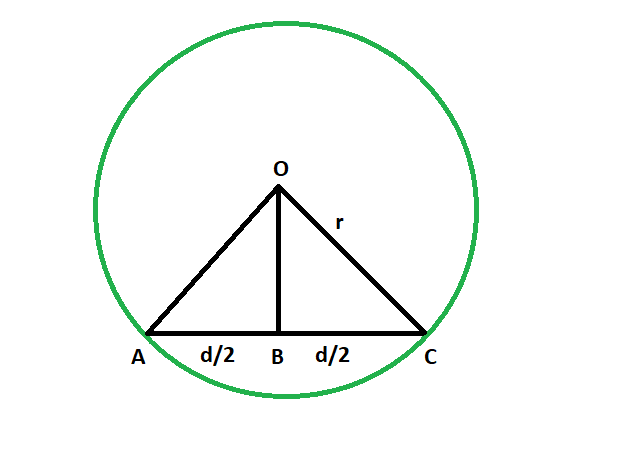Open in App
Not now

# Shortest distance from the center of a circle to a chord

• Last Updated : 26 Nov, 2022

Given a circle which has a chord inside it. The length of the chord and the radius of the circle are given. The task is to find the shortest distance from the chord to the center.
Examples:

```Input: r = 4, d = 3
Output: 3.7081

Input: r = 9.8, d = 7.3
Output: 9.09492```Approach

• We know that the line segment that is dropped from the center on the chord bisects the chord. The line is the perpendicular bisector of the chord, and we know the perpendicular distance is the shortest distance, so our task is to find the length of this perpendicular bisector.
• let radius of the circle = r
• length of the chord = d
• so, in triangle OBC,
from Pythagoras theorem
OB^2 + (d/2)^2 = r^2
so, OB = √(r^2 – d^2/4)
• So,Below is the implementation of the above approach:

## C++

 `// C++ program to find` `// the shortest distance from` `// chord to the center of circle` `#include ` `using` `namespace` `std;`   `// Function to find the shortest distance` `void` `shortdis(``double` `r, ``double` `d)` `{` `    ``cout << ``"The shortest distance "` `         ``<< ``"from the chord to center "` `         ``<< ``sqrt``((r * r) - ((d * d) / 4))` `         ``<< endl;` `}`   `// Driver code` `int` `main()` `{` `    ``double` `r = 4, d = 3;` `    ``shortdis(r, d);` `    ``return` `0;` `}`

## Java

 `// Java program to find ` `// the shortest distance from ` `// chord to the center of circle ` `class` `GFG` `{` `    `  `// Function to find the shortest distance ` `static` `void` `shortdis(``double` `r, ``double` `d) ` `{ ` `    ``System.out.println(``"The shortest distance "` `        ``+ ``"from the chord to center "` `        ``+ (Math.sqrt((r * r) - ((d * d) / ``4``)))); ` `} `   `// Driver code ` `public` `static` `void` `main(String[] args)` `{` `    ``double` `r = ``4``, d = ``3``; ` `    ``shortdis(r, d); ` `}` `}`   `/* This code contributed by PrinciRaj1992 */`

## Python3

 `# Python program to find ` `# the shortest distance from ` `# chord to the center of circle `   `# Function to find the shortest distance ` `def` `shortdis(r, d): ` `    ``print``(``"The shortest distance "``,end``=``"");` `    ``print``(``"from the chord to center "``,end``=``"");` `    ``print``(((r ``*` `r) ``-` `((d ``*` `d) ``/` `4``))``*``*``(``1``/``2``));`   `# Driver code ` `r ``=` `4``;` `d ``=` `3``; ` `shortdis(r, d); `   `# This code has been contributed by 29AjayKumar`

## C#

 `// C# program to find ` `// the shortest distance from ` `// chord to the center of circle ` `using` `System;`   `class` `GFG ` `{ ` `    `  `    ``// Function to find the shortest distance ` `    ``static` `void` `shortdis(``double` `r, ``double` `d) ` `    ``{ ` `        ``Console.WriteLine(``"The shortest distance "` `            ``+ ``"from the chord to center "` `            ``+ (Math.Sqrt((r * r) - ((d * d) / 4)))); ` `    ``} ` `    `  `    ``// Driver code ` `    ``public` `static` `void` `Main() ` `    ``{ ` `        ``double` `r = 4, d = 3; ` `        ``shortdis(r, d); ` `    ``} ` `} `   `// This code is contributed by AnkitRai01`

## PHP

 ``

## Javascript

 ``

Output

```The shortest distance from the chord to center 3.7081
```

Time Complexity: O(1)

Auxiliary Space: O(1)

My Personal Notes arrow_drop_up
Related Articles